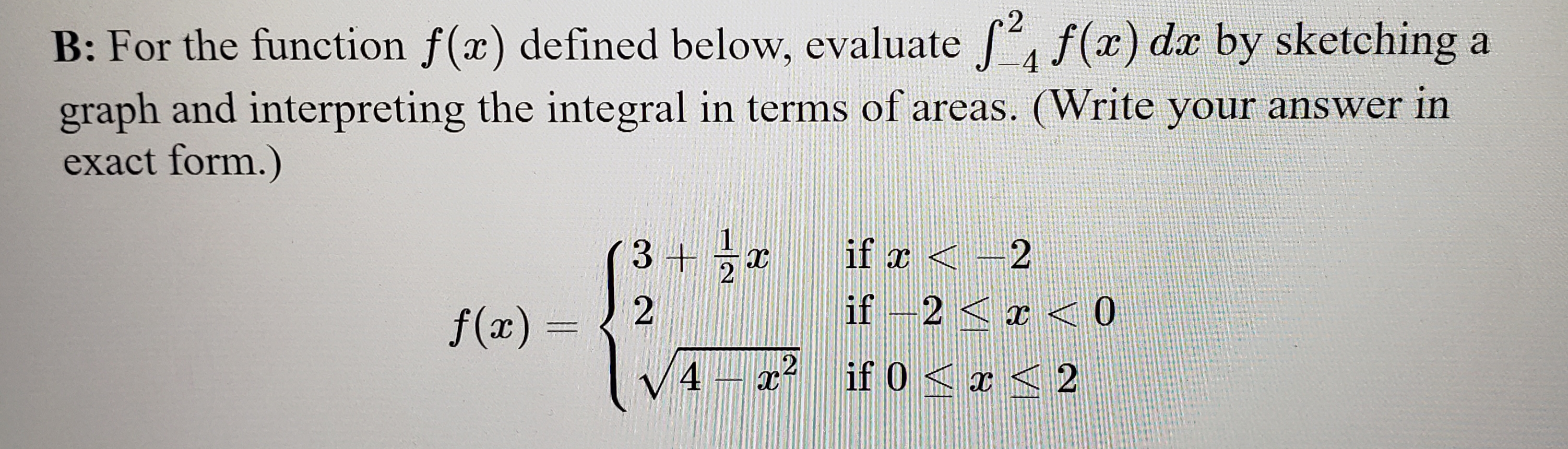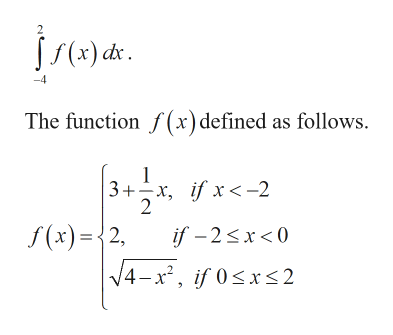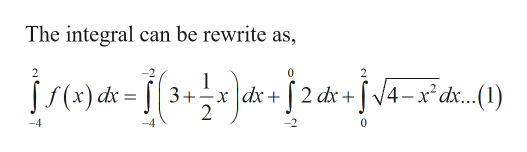# 2f(x) dr by sketching aB: For the function f(x) defined below, evaluate4graph and interpreting the integral in terms of areas. (Write your answer inexact form.)3 +2if x 2if -2< x< 02f(x) =2 if 0 < 24

Question
57 viewshelp_outlineImage Transcriptionclose2 f(x) dr by sketching a B: For the function f(x) defined below, evaluate 4 graph and interpreting the integral in terms of areas. (Write your answer in exact form.) 3 +2 if x 2 if -2< x< 0 2 f(x) = 2 if 0 < 2 4 fullscreen
check_circle

Step 1

Given:help_outlineImage Transcriptionclose2 J(x) dx The functionf (x)defined as follows 3+x, if x<-2 2 f(x)2, if-2sx<0 = V4-x2, if 0
Step 2

Calculation:

Step 3help_outlineImage TranscriptioncloseThe integral can be rewrite as, 2 1 JS(x) dx=3+. x dx+2 dr +V4-x2dx...(1) 2 -2 0 -4 fullscreen

### Want to see the full answer?

See Solution

#### Want to see this answer and more?

Solutions are written by subject experts who are available 24/7. Questions are typically answered within 1 hour.*

See Solution
*Response times may vary by subject and question.
Tagged in

### Integration